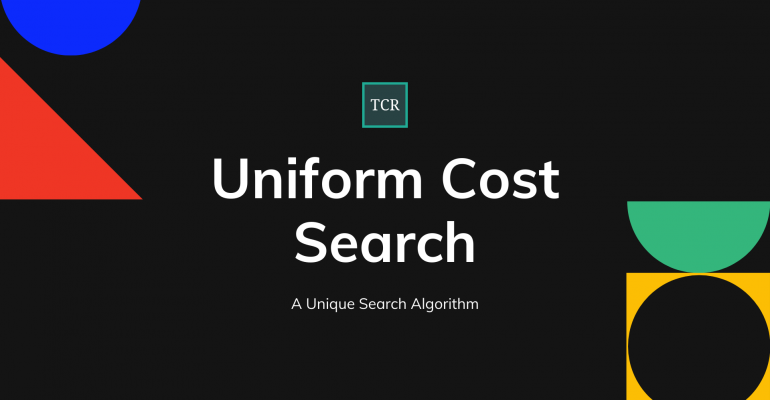Thanks to theidioms.com

### Infosys Tech Scholars Scholarship## Uniform Cost Search – Algorithm, Benefits, and Comparison with Djikstra# Uniform Cost Search – Algorithm, Benefits, and Comparison with Djikstra

Greetings! Some links on this site are affiliate links. That means that, if you choose to make a purchase, The Click Reader may earn a small commission at no extra cost to you. We greatly appreciate your support!

Uniform Cost Search is a technique employed to change the position of a directed weighted search space from a start node to one of the ending nodes with a minimum cumulative cost.

## Uniform Cost Search Vs Dijkstra’s algorithm

Dijkstra’s and uniform cost algorithm is known to solve the shortest path problem with similar time complexity. Both have the same code structures. Also, we use the same formula dist[v] = min (dist[v], dist[u] + w (u,  v)) to update the distance value of each vertex.

The primary difference between these two techniques is how we classify vertices in Q. In Dijkstra’s technique, you initialize Q and every vertex in G; hence, we can say that Dijkstra’s technique primarily uses detailed graphs to realize all vertices and edges.

On the other hand, the uniform cost search algorithm begins with the root vertex and continually passes over the necessary graph parts; hence, it is applicable for explicit and implicit graphs.

As for the single-pair shortest path problem, Dijkstra’s technique has more storage requirements because you ought to store the entire graph in memory. On the contrary, the uniform cost search technique mainly stores the source vertex and stops broadening once you get to the end vertex, so the uniform cost search algorithm may only store a partial graph in the end.

Although the two techniques have similar time complexity on the single-pair shortest path situation, Dijkstra’s technique can be more time-consuming than the other because of memory requirements.

When you implement Q as a min-heap priority queue, the two queue operation takes O(logn) time, where n is the number of vertices in Q. Dijkstra’s algorithm puts all vertices Q at the beginning. As for a large graph, its vertices will create a big overhead when performing operations Q, but the uniform cost search starts with one vertex and subsequently includes other vertices at the time of building the path, so you’ll be working on a small number of vertex whenever you do priority queue operations.

## Pros and cons of Uniform Cost Search

Pro: It will help you find the path with the most reduced cumulative cost in a weighted graph having a different cost associated with each of its edges from the start node to the end node. Due to the least path being followed at each state, It is considered an optimal solution.

Con: You are required to keep the empty list sorted as priorities in the priority queue need to be protected. The storage requirement is hilariously large. You are to stop it from going on an infinite loop as it considers every possible path going from the start node to the end node.

## Conclusion

Uniform Cost Search is a unique search algorithm solution to find the path from the root node to the destination node with the lowest cumulative cost in a weighted search space where every node has a unique traversal cost. It is the same as Heuristic Search; however, no Heuristic information is stored, implying h=0.Do you want to learn Python, Data Science, and Machine Learning while getting certified? Here are some best selling Datacamp courses that we recommend you enroll in:

1. Introduction to Python (Free Course) - 1,000,000+ students already enrolled!
2. Introduction to Data Science  in Python- 400,000+ students already enrolled!
3. Introduction to TensorFlow for Deep Learning with Python - 90,000+ students already enrolled!
4. Data Science and Machine Learning Bootcamp with R - 70,000+ students already enrolled!